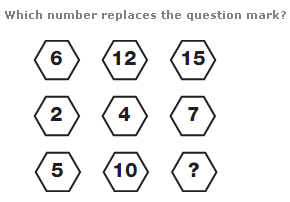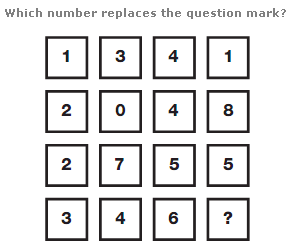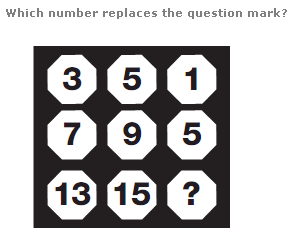# Puzzles - Number puzzles

### Exercise :: Number puzzlesAnswer : 13 Explanation : Working in rows, from left to right, double the left hand number to get the middle number, and add 3 to this to get the right hand number.Answer : 2 Explanation : Split the diagram into two halves vertically, consisting of 2 columns of 2 squares each, and read each 2 adjoining squares as a complete 2 digit number. Numbers follow the sequence of multiples of 7, subtracting 1 each time.Answer : 11 Explanation : Working through the diagram in columns, add 4 to the top number to give the middle one, and add 6 to this number to give the lowest one.